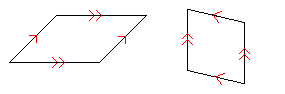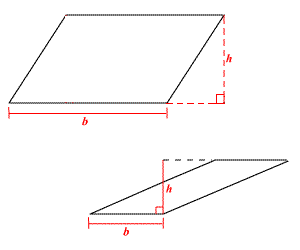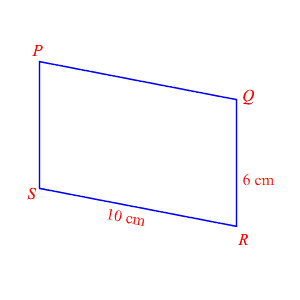# Parallelograms: Area and Perimeter

A parallelogram is a quadrilateral in which both pairs of opposite sides are parallel .It can be shown that opposite sides of parallelogram must be congruent , that opposite angles are also congruent , and that consecutive angles are supplementary .

The area $A$ of a parallelogram is given by the formula

$A=bh$ ,

where $b$ is the length of one base and $h$ is the height. (The figure below shows how to find the height of a parallelogram.)The perimeter of a parallelogram is the sum of the lengths of its four sides.

For example, in the figure below, if $PQRS$ is a parallelogram, then we know opposite sides are congruent, so $PQ=10$ and $PS=6$ .Therefore, the perimeter is $6+10+6+10$ or $32$ cm.